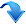3.5 g. Thermodynamic properties of alkali silicate glasses - comparison of experiment and calculations (N. Zotov)

The heat capacity of silicate glasses and melts is of increasing importance in petrology and volcanology, as calculations involving the physical and chemical properties of magmas become more quantitative. Furthermore, heat capacity data are also important for theoretical reasons because of the close relationship between the thermodynamic and vibrational properties of condensed matter phases.

A great number of heat capacity measurements of silicate glasses and melts are published in the literature. They have been used to derive empirical equations for the calculation of the heat capacity and relative enthalpies as a function of temperature and chemical composition assuming ideal-mixing of the partial molar heat capacities of oxide components.

Calculations of the thermodynamic properties using realistic atomistic models have been reported only recently for liquids in the system NaAlSiO4 - SiO2 at very high temperatures (2500 - 4000 K) using molecular dynamics simulations.

We have performed first calculations of the specific heat at constant volume (Cv), the specific heat at constant pressure (Cp) and the entropy (S) below the glass transition temperature (Tg) for several alkali silicate glasses with composition of SiO2, Na2O·4SiO2 (NS4), Na2O·2SiO2 (NS2) and Na2O·SiO2 (NS1) using computer models involving molecular dynamics and/or reverse Monte Carlo simulations. The models contain from 234 to 1080 atoms. The vibrational density of states (VDOS) of the models was calculated using valence type harmonic potential and direct diagonalization of the dynamical matrix. Cv was calculated using the standard statistical-mechanics relation between Cv and VDOS, assuming completely vibrational origin of the heat capacity. Cp was then calculated as Cp(T) = Cv(T) +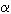2KVT, where the temperature dependences of the expansion coefficient, the bulk modulus K and the molar volume V were taken from the literature.

Figures 3.5-9 and 3.5-10 compare our calculated heat capacities of SiO2 and NS2 glasses, respectively, to those experimentally measured by different authors. The calculated Cp for amorphous SiO2 is in excellent agreement with experiment in a wide temperature range (30 - 1200 K). For sodium silicate glasses the calculated Cp close to Tg remains below the Dulong-Petit limit and the discrepancy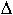Cp = Cpexp - Cpcal increases with increasing degree of depolymerization.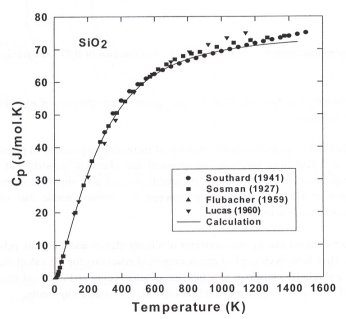Fig. 3.5-9: Temperature dependence of the calculated (solid line) and experimental heat capacities of amorphous SiO2.

Detailed analysis of the partial atomic heat capacities derived from the corresponding partial VDOS shows that: (i) The bridging oxygens (BO) give the largest contribution to Cv; with increasing degree of depolymerization the BO contribution decreases linearly at the expense of the contributions of non-bridging oxygens (NBO) and Na contributions. This result explains quantitatively the experimentally observed increase of the total heat capacity with decreasing concentration of the SiO2 component; (ii) The Na-O vibrations contribute significantly to the heat capacity at low temperatures (T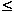100 K) but are practically independent of T at high temperatures; (iii) the thermodynamic properties of sodium silicate glasses are only weakly dependent on the actual Q-species distribution and the amount of medium-range order; (iv) the anharmonic contributions to the heat capacity at high temperatures increase with increasing degree of depolymerization.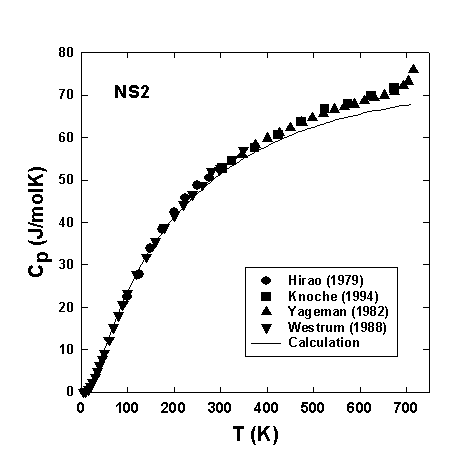Fig. 3.5-10: Temperature dependence of calculated (solid line) and experimental heat capacities of sodium disilicate glass on temperature.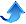Bayerisches Geoinstitut, Universität Bayreuth, 95440 Bayreuth, Deutschland
Tel: +49-(0) 921 55 3700 / 3766, Fax: +49-(0) 921 55 3769, E-mail: bayerisches.geoinstitut(at)uni-bayreuth.de